# Distributive lattice

(diff) ← Older revision | Latest revision (diff) | Newer revision → (diff)

A lattice in which the equationholds. This equation is equivalent to both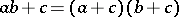and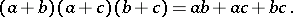Distributive lattices are characterized by the fact that all their convex sublattices can occur as congruence classes. Any distributive lattice is isomorphic to a lattice of (not necessarily all) subsets of some set. An important special case of such lattices are Boolean algebras (cf. Boolean algebra). For any finite setin a distributive lattice the following equalities are valid:and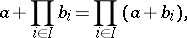as well as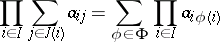and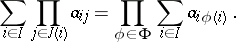Here the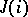are finite sets andis the set of all single-valued functionsfrominto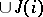such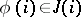for each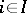. In a complete lattice the above equations also have a meaning if the setsandare infinite. However, they do not follow from the distributive law. Distributive complete lattices (cf. Complete lattice) which satisfy the two last-mentioned identities for all setsandare called completely distributive.

How to Cite This Entry:
Distributive lattice. Encyclopedia of Mathematics. URL: http://encyclopediaofmath.org/index.php?title=Distributive_lattice&oldid=19143
This article was adapted from an original article by L.A. Skornyakov (originator), which appeared in Encyclopedia of Mathematics - ISBN 1402006098. See original article